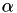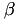Simpact Cyan Population based event driven simulation using mNRM
GslRandomNumberGenerator Class Reference

This class allows you to generate random numbers, and uses the GNU Scientific Library for this. More...

#include <gslrandomnumbergenerator.h>

Public Member Functions

GslRandomNumberGenerator ()
Initialize the random number generator with a random seed, A specific seed can still be forced by setting the MNRM_DEBUG_SEED environment variable (can be useful for testing purposes). More...

GslRandomNumberGenerator (int seed)
Initialize the random number generator with a specific seed. More...

double pickRandomDouble ()
Generate a random floating point number in the interval [0,1]. More...

int pickRandomInt (int min, int max)
Chooses a random number from min to max (both are included). More...

unsigned int pickPoissonNumber (double lambda)
Chooses a random number according to the Poisson distribution with parameter lambda. More...

double pickGaussianNumber (double mean, double sigma)
Picks a random number from the gaussian distribution with parameters mean and sigma. More...

double pickBetaNumber (double a, double b)
Pick a random number from a beta distribution with a and b as values forandrespectively. More...

double pickWeibull (double lambda, double kappa)
Picks a random number from a Weibull distribution with specified parameters. More...

double pickWeibull (double lambda, double kappa, double ageMin)
Picks a random number from a distribution which has a Weibull shape with specified parameters above ageMin, and which is zero below that age. More...

double pickLogNorm (double zeta, double sigma)
Picks a random number from a log-normal distribution with parameters zeta and sigma. More...

double pickGamma (double a, double b)
Picks a random number from the gamma distribution with a and b defined as in the formula prob(x) = x^(a-1)*exp(-x/b)/(b^a * Gamma(a)) . More...

std::pair< double, double > pickBivariateGaussian (double muX, double muY, double sigmaX, double sigmaY, double rho)
Picks a random number from a two dimensional gaussian distribution with specified parameters (rho is the correlation coefficient). More...

Detailed Description

This class allows you to generate random numbers, and uses the GNU Scientific Library for this.

Constructor & Destructor Documentation

 GslRandomNumberGenerator::GslRandomNumberGenerator ( )

Initialize the random number generator with a random seed, A specific seed can still be forced by setting the MNRM_DEBUG_SEED environment variable (can be useful for testing purposes).

 GslRandomNumberGenerator::GslRandomNumberGenerator ( int seed )

Initialize the random number generator with a specific seed.

Member Function Documentation

 double GslRandomNumberGenerator::pickBetaNumber ( double a, double b )

Pick a random number from a beta distribution with a and b as values forandrespectively.

 std::pair< double, double > GslRandomNumberGenerator::pickBivariateGaussian ( double muX, double muY, double sigmaX, double sigmaY, double rho )

Picks a random number from a two dimensional gaussian distribution with specified parameters (rho is the correlation coefficient).

 double GslRandomNumberGenerator::pickGamma ( double a, double b )

Picks a random number from the gamma distribution with a and b defined as in the formula prob(x) = x^(a-1)*exp(-x/b)/(b^a * Gamma(a)) .

 double GslRandomNumberGenerator::pickGaussianNumber ( double mean, double sigma )

Picks a random number from the gaussian distribution with parameters mean and sigma.

 double GslRandomNumberGenerator::pickLogNorm ( double zeta, double sigma )

Picks a random number from a log-normal distribution with parameters zeta and sigma.

 unsigned int GslRandomNumberGenerator::pickPoissonNumber ( double lambda )

Chooses a random number according to the Poisson distribution with parameter lambda.

 double GslRandomNumberGenerator::pickRandomDouble ( )

Generate a random floating point number in the interval [0,1].

 int GslRandomNumberGenerator::pickRandomInt ( int min, int max )

Chooses a random number from min to max (both are included).

 double GslRandomNumberGenerator::pickWeibull ( double lambda, double kappa )

Picks a random number from a Weibull distribution with specified parameters.

 double GslRandomNumberGenerator::pickWeibull ( double lambda, double kappa, double ageMin )

Picks a random number from a distribution which has a Weibull shape with specified parameters above ageMin, and which is zero below that age.

The documentation for this class was generated from the following files: